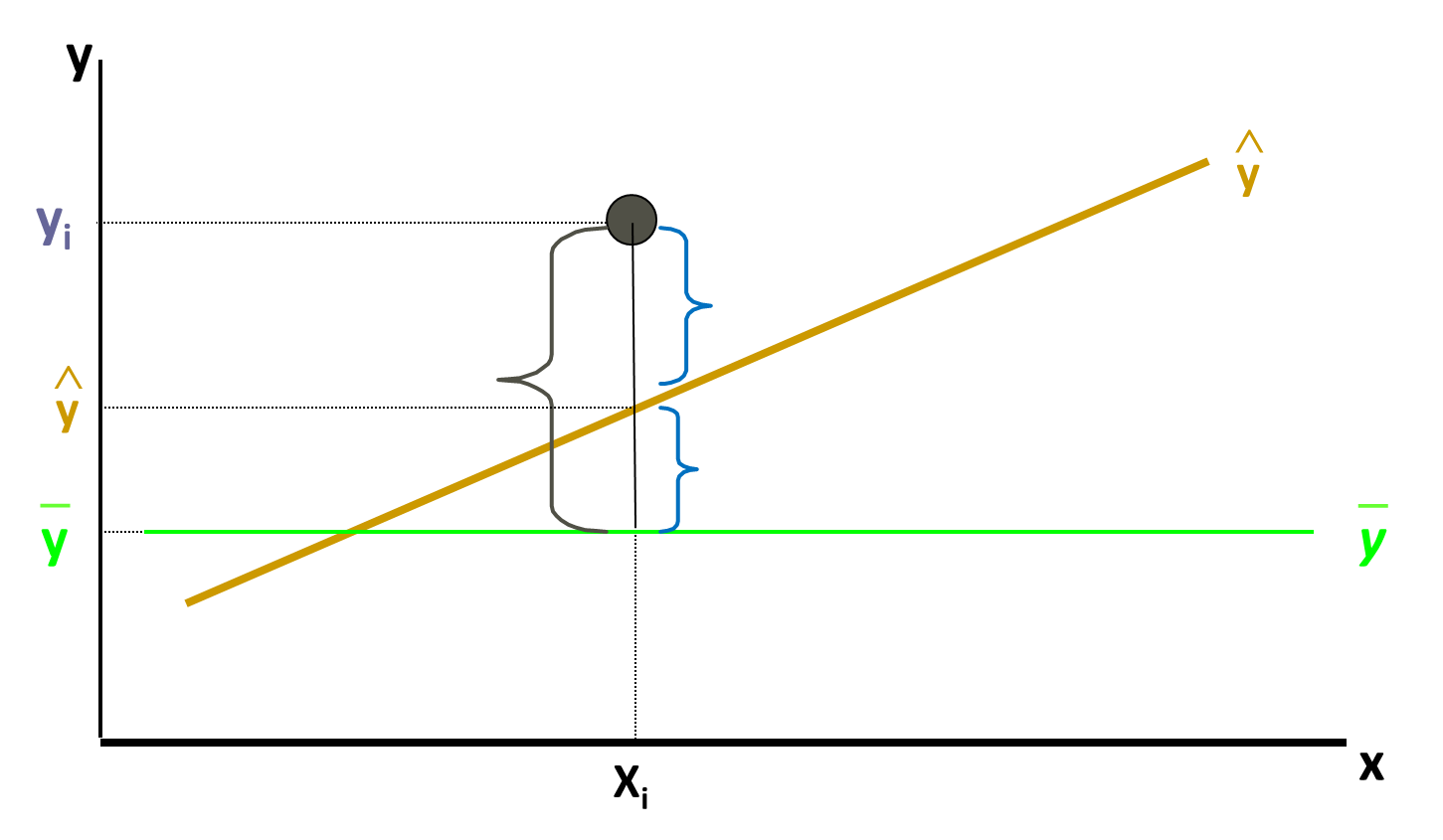• No products in the cart.

# 203.1.6 How good is my Regression Line in R

##### Mathematics behind a good regression line fitting.

In the previous section we discussed about   Practice : Regression Line Fitting in R

#### How good is my regression line?

What is the proof that the regression line that is built is the best one? What if someone challenges our regression line? What is the accuracy of our model? There is a simple way to prove that the regression line that we had drawn is the best one. From the given dataset of Air passengers, we can see that if the promotional budget of 517356 is spent then numbers of passengers are 37824. So for checking the accuracy of the regression line that we had drawn, we can re-frame the question as “Predict the number of passengers if the promotional budget is 517356? For the data we already know the answer but still we did the estimation for the numbers of passengers if the Promotional budget spent is of 517356 and the estimation value we got for number of passengers is 37231.21, which is pretty good but yes there are still some errors because the actual value is 37824 and predicted value is 37231.21.

When do we say that our regression line is perfect?

The regression line is perfect when the estimated value and the predicted value both are exactly same, and the difference or deviation between them is an error. Once again to know how well is the regression line that we had drawn, just take a point from the dataset that is already present in the historical data, now just submit the value of the variable X in the regression line then the variable Y’s prediction value will be estimated. If the regression line is better fit then we can except that Y predicted is the value which is exactly same as the value of Y in the given datasets or we can say Predicted value of Y – Actual value of Y should be zero. If we repeat this for multiple times we can observe that some deviations are positive some are negative this means some time the value of prediction can be higher than the actual value and in some cases the value of prediction can be lower than the actual value. For the best line, Y predicted – Y actual should be zero. So for a good regression line the overall deviations should be less. Since some deviations are positive and some are negative, so we can take sum of square of all such deviations. For a given regression line if the sum of all the square of such deviations is taken in consideration then error should be really less. So while comparing two regression lines look at the sum of the squares of errors of both regression lines, the regression line that makes fewer errors is the better line.

SSE=(yy^)2

IF SSE (sum of squared error) is near to zero, then we have a good model. Most of the times standalone SSE is not sufficient. For example, if SSE value is 100 so this value is near to 0 or is it far from 0 actually we can’t tell because the value of SSE seems very less if the value of Y is thousands or millions ,so the deviation of 100 according to the SSE value is not really high. But if we are working or dealing with fraction or decimals the value of SSE 100 that is really high, so to conclude that if the SSE is having high value or not the variable Y should bring into the picture. So how do we find that how well or correct is our regression line? To answer these just understand the following derivation which will tell us the goodness of fit of our regression line.

Error Sum of squares (SSE- Sum of Squares of error)

SSE=(yy^)2

This is sum of squares of errors this should be least for given regression line.

Total Variance in Y (SST- Sum of Squares of Total)

SST=(yy¯)2

We can rewrite as,

SST=(yy^+y^y¯)2
SST=(yy^+y^y¯)2
SST=(yy^)2+(y^y¯)2
SST=SSE+(y^y¯)2
SST=SSE+SSR

### Explained and Unexplained VariationSo, total variance in Y is divided into two parts, + Variance that can’t be explained by x (error): SSE + Variance that can be explained by x, using regression : SSR

SST=SSE+SSR
TotalsumofSquares=SumofSquaresError+SumofSquaresRegression
SST=(yy¯)2SSE=(yy^)2SSR=(y^y¯)2

In next session we will figure out Rsquared which a statistical measure of closeness of data points to the fitted regression line.

20th June 2017